##### The Japanese Bonsai specialist
Direct order Contact Help / Services Newsletter# Acer matsumurae seeds inazuma

Graines érables japonais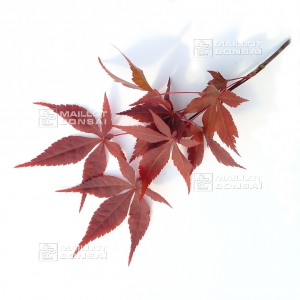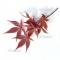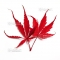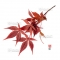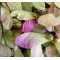ref. : 66

6,00

Available quantity : 19Order

###### Description

Leaves: brownish crimson in spring, brown in summer and reddish orange in autumn.

Bag of about 60 seeds. Harvested on november 2021.

#seeds 5.5 #acer 4.4 #matsumurae 3 #inazuma 2.7 #more 2.4 #harvested 1.9 #brownish 1.8 #november 1.8 #reddish 1.7 #crimson 1.7

Formule
(( ROUND((CHAR_LENGTH(b.article_nom)-CHAR_LENGTH(REPLACE(b.article_nom, 'seeds', '')))/LENGTH('seeds')) + ROUND((CHAR_LENGTH(b.article_description)-CHAR_LENGTH(REPLACE(b.article_description, 'seeds', '')))/LENGTH('seeds')) ) * 3.5) + (( ROUND((CHAR_LENGTH(b.article_nom)-CHAR_LENGTH(REPLACE(b.article_nom, 'matsumurae', '')))/LENGTH('matsumurae')) + ROUND((CHAR_LENGTH(b.article_description)-CHAR_LENGTH(REPLACE(b.article_description, 'matsumurae', '')))/LENGTH('matsumurae')) ) * 3) + (( ROUND((CHAR_LENGTH(b.article_nom)-CHAR_LENGTH(REPLACE(b.article_nom, 'inazuma', '')))/LENGTH('inazuma')) + ROUND((CHAR_LENGTH(b.article_description)-CHAR_LENGTH(REPLACE(b.article_description, 'inazuma', '')))/LENGTH('inazuma')) ) * 2.7) + (( ROUND((CHAR_LENGTH(b.article_nom)-CHAR_LENGTH(REPLACE(b.article_nom, 'acer', '')))/LENGTH('acer')) + ROUND((CHAR_LENGTH(b.article_description)-CHAR_LENGTH(REPLACE(b.article_description, 'acer', '')))/LENGTH('acer')) ) * 2.4) + (( ROUND((CHAR_LENGTH(b.article_nom)-CHAR_LENGTH(REPLACE(b.article_nom, 'harvested', '')))/LENGTH('harvested')) + ROUND((CHAR_LENGTH(b.article_description)-CHAR_LENGTH(REPLACE(b.article_description, 'harvested', '')))/LENGTH('harvested')) ) * 1.9) + (( ROUND((CHAR_LENGTH(b.article_nom)-CHAR_LENGTH(REPLACE(b.article_nom, 'brownish', '')))/LENGTH('brownish')) + ROUND((CHAR_LENGTH(b.article_description)-CHAR_LENGTH(REPLACE(b.article_description, 'brownish', '')))/LENGTH('brownish')) ) * 1.8) + (( ROUND((CHAR_LENGTH(b.article_nom)-CHAR_LENGTH(REPLACE(b.article_nom, 'november', '')))/LENGTH('november')) + ROUND((CHAR_LENGTH(b.article_description)-CHAR_LENGTH(REPLACE(b.article_description, 'november', '')))/LENGTH('november')) ) * 1.8) + (( ROUND((CHAR_LENGTH(b.article_nom)-CHAR_LENGTH(REPLACE(b.article_nom, 'crimson', '')))/LENGTH('crimson')) + ROUND((CHAR_LENGTH(b.article_description)-CHAR_LENGTH(REPLACE(b.article_description, 'crimson', '')))/LENGTH('crimson')) ) * 1.7) + (( ROUND((CHAR_LENGTH(b.article_nom)-CHAR_LENGTH(REPLACE(b.article_nom, 'reddish', '')))/LENGTH('reddish')) + ROUND((CHAR_LENGTH(b.article_description)-CHAR_LENGTH(REPLACE(b.article_description, 'reddish', '')))/LENGTH('reddish')) ) * 1.7) + (( ROUND((CHAR_LENGTH(b.article_nom)-CHAR_LENGTH(REPLACE(b.article_nom, 'autumn', '')))/LENGTH('autumn')) + ROUND((CHAR_LENGTH(b.article_description)-CHAR_LENGTH(REPLACE(b.article_description, 'autumn', '')))/LENGTH('autumn')) ) * 1.6)

## Secure payment## Delivery

Our logistic partners :04 74 55 23 48
Pépinière MAILLOT-BONSAÏ
Le Bois Frazy
01990 RELEVANT - FRANCE
on appointmentThe Japanese Bonsai specialist
If you like Maillot Bonsaï, share on  facebook

Pensez à venir nous rendre visite sur rendez vous. 04 74 55 23 48 merci.

L'équipe Maillot bonsaï vous souhaite ses meilleurs vœux de bonheur, de santé et de réussite pour cette nouvelle année. C'est l'occasion pour nous de vous dire un IMMENSE MERCI d'avoir été à nos côtés cette année.

2023, c'est 365 jours où nous serons présents pour vous aider, vous guider et répondre à vos questions. Plus que jamais, nous continuerons à vous accompagner et à vous proposer des produits de qualité.   Cette nouvelle année sera riche en nouveautés, alors restez connecté pour ne rien rater !

Guy Maillot

OK